# 1 & 2 persons - Page 2

Viewing mode
29 results
• Slopes access :
• 50 -300m to the slopes
• Rooms :
• Studio
• Total surface (sq.m) :
• 19 sq.m
•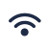•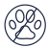• Slopes access :
• 50 -300m to the slopes
• Rooms :
• 2 rooms
• Total surface (sq.m) :
• 40 sq.m
•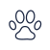•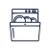•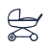• Slopes access :
• 50 -300m to the slopes
• Rooms :
• Studio
• Total surface (sq.m) :
• 20 sq.m
•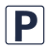••• Slopes access :
• 50 -300m to the slopes
• Rooms :
• Studio
• Total surface (sq.m) :
• 18 sq.m
••• Rooms :
• Studio
• Studio + cabin(s)
• Total surface (sq.m) :
• 15 sq.m
••• Slopes access :
• 50 -300m to the slopes
• Rooms :
• Studio
• Studio + cabin(s)
• Total surface (sq.m) :
• 19 sq.m
•••• Slopes access :
• ski-in (-50 m)
• Rooms :
• Studio
• Total surface (sq.m) :
• 16 sq.m
••• Slopes access :
• ski-in (-50 m)
• Rooms :
• Studio
• Total surface (sq.m) :
• 20 sq.m
•••• Slopes access :
• ski-in (-50 m)
• Total surface (sq.m) :
• 35 sq.m
•••